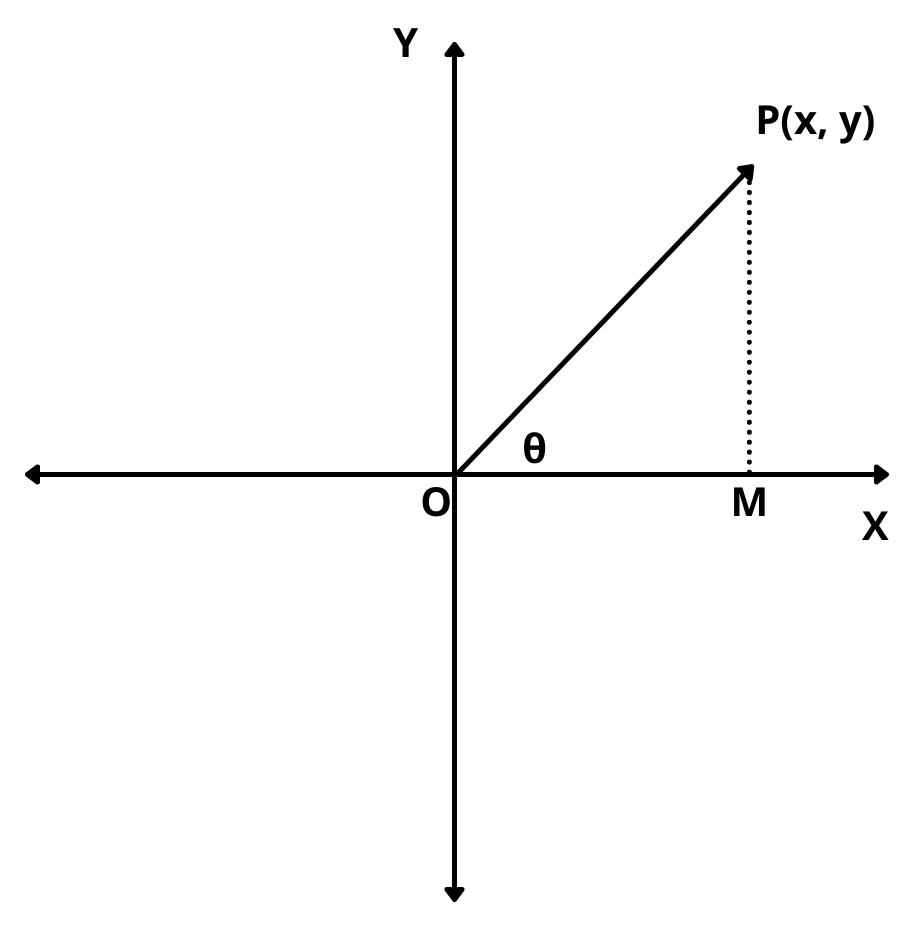# Amplitude of a Complex Number

Here you will learn how to find argument or amplitude of a complex number with examples.

Let’s begin –

## Amplitude of a Complex Number (Argument of Complex Number)

Let z = x + iy, Then,

The angle $$\theta$$ which OP makes with the positive direction of x-axis in anticlockwise sense is called the argument or amplitude of complex number z.It is denoted by arg(z) or amp(z).

From Figure, we have

$$tan\theta$$ = $$PM\over OM$$ = $$y\over x$$ = $$Im (z)\over Re (z)$$

$$\implies$$ $$\theta$$ = $$tan^{-1}({y\over x}$$)

This angle $$\theta$$ has infinitely many values differing by multiples of $$2\pi$$. The unique value of $$\theta$$ such that -$$\pi$$ < $$\theta$$ $$\le$$ $$\pi$$ is called the principal value of the amplitude or principal argument.

In order to find the argument of a complex number z = x + iy for different quadrants, we use the following algorithm.

#### Algorithm :

1). Find the value of $$tan^{-1}| y/x |$$ lying between 0 and $$\pi/2$$. Let it be $$\alpha$$.

2). Determine in which quadrant the point P(x, y) belongs.

(i) If P(x, y ) belongs to the first quadrant, then arg(z) = $$\alpha$$.

(ii) If P(x, y ) belongs to the second quadrant, then arg(z) = $$\pi – \alpha$$.

(iii) If P(x, y ) belongs to the third quadrant, then arg(z) = -($$\pi – \alpha$$) or $$\pi + \alpha$$.

(iv) If P(x, y ) belongs to the fourth quadrant, then arg(z) = -$$\alpha$$ or $$2\pi – \alpha$$.

Example : Find the argument of the following complex numbers.

(i) $$1 + i\sqrt{3}$$

(ii) $$-2 + 2i\sqrt{3}$$

(iii) $$-\sqrt{3} – i$$

(iv) $$2\sqrt{3} – 2i$$

Solution

(i) Let  z = $$1 + i\sqrt{3}$$ and let $$\alpha$$ be the acute angle given by

$$\alpha$$ = $$tan^{-1}|{Im(z)\over Re(z)}|$$ = $$tan^{-1}|{\sqrt{3}\over 1}|$$

$$\implies$$ $$\alpha$$ = $$\pi\over 3$$

Since, Re(z) > 0 and Im(z) > 0. So, it lies in first quadrant

$$\therefore$$  arg(z) = $$\alpha$$ = $$\pi\over 3$$

(ii) Let  z = $$-2 + 2i\sqrt{3}$$ and let $$\alpha$$ be the acute angle given by

$$\alpha$$ = $$tan^{-1}|{Im(z)\over Re(z)}|$$ = $$tan^{-1}|{2\sqrt{3}\over -2}|$$

$$\implies$$ arg(z) = $$\alpha$$ = $$\pi\over 3$$

Since, Re(z) < 0 and Im(z) > 0. So, it lies in second quadrant

$$\therefore$$ arg(z) = $$\pi – \alpha$$ = $$\pi$$ – $$\pi\over 3$$ = $$2\pi\over 3$$

(iii) Let  z = $$-\sqrt{3} – i$$ and let $$\alpha$$ be the acute angle given by

$$\alpha$$ = $$tan^{-1}|{Im(z)\over Re(z)}|$$ = $$tan^{-1}|{-1\over -\sqrt{3}}|$$

$$\implies$$ $$\alpha$$ = $$\pi\over 6$$

Since, Re(z) < 0 and Im(z) < 0. So, it lies in third quadrant

$$\therefore$$  arg(z) = -($$\pi – \alpha$$) = -($$\pi$$ – $$\pi\over 6$$) = $$-5\pi\over 6$$

(iv) Let  z = $$2\sqrt{3} – 2i$$ and let $$\alpha$$ be the acute angle given by

$$\alpha$$ = $$tan^{-1}|{Im(z)\over Re(z)}|$$ = $$tan^{-1}|{-2\over 2\sqrt{3}}|$$

$$\implies$$ $$\alpha$$ = $$\pi\over 6$$

Since, Re(z) > 0 and Im(z) < 0. So, it lies in fourth quadrant

$$\therefore$$  arg(z) = -$$\alpha$$ = -$$\pi\over 6$$